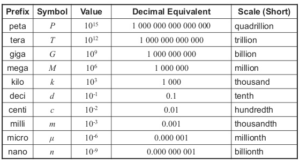# Prefixes

0
6060

It is clear that it is not enough to use only one measuring unit for a particular physical size. So offten, besides basic units, larger and smaller units of measure are introduced.

To indicate these units, we use prefixes that express how many times a certain unit is larger or smaller than a basic or derived unit of measurement.

Prefixes are used for easier recording since it is complicated to write too large or too small numbers with a lot of zeros. Thus, when we measure some of the magnitudes such as space distance in the universe or the size of the molecules or atoms and subatomic particles, it is easier to use prefixes.

A prefix “deci” means that the given size is ten times smaller than the unit in which it stands.
The prefix “deca” means that the given size is ten times larger than the unit in which it stands.
The prefix “centi” means that the given data is hundreds of times smaller than the unit in which it stands.
The prefix “hecto” means that the data is a hundred times greater than the unit in which it stands.
The prefix “milli” means that the given size is a thousand times smaller than the measuring unit it is standing with.
The prefix “kilo” means that the amount of data is a thousand times greater than the unit in which it stands.

What do the prefixes mean?
We all use the computers today and we all heard of the word “byte”. Byte is a unit of measurement for the amount of data in the computer.
The same prefixes are used: kilo, mega and giga, which because they are binary rather than a decade, do not have the same value as prefixes for our measurement units, but their values are approximately the same.

See table showing the unit for length. All other basic and derived units of measurement have larger and smaller units of measure.
We can see from the following table the prefixes for smaller and larger units.SHARE
Previous articleInternational System of Units (SI)
Next articleTHE BAROMETER FABLE